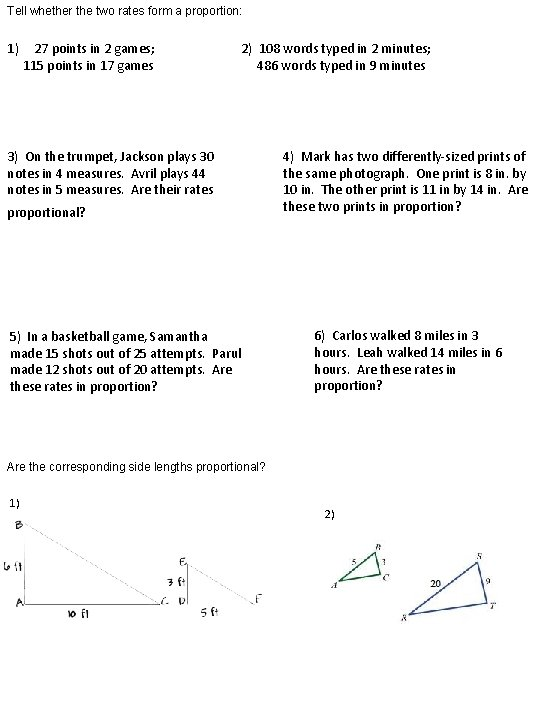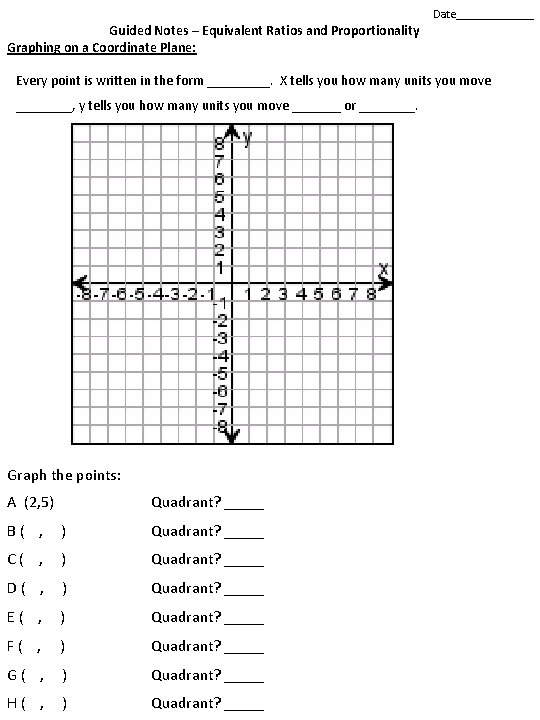# Guided Notes Equivalent Ratios and Proportionality Date Two

• Slides: 4Guided Notes – Equivalent Ratios and Proportionality Date ________ Two ratios are ________ if they form equal _______ or have equal unit rates. Testing whether the ratios form a proportion can be done in three ways. 1. Find the __________ 2. _________fractions 3. _________ multiply Determine if the ratios form a proportion: 1) 2) 4) 3) Determine if x and y form a proportional relationship from the table: 1) x 3 4 5 6 y 9 11 13 15 2) x y Unit Rate _____ 3) 2 3 4 2. 5 5 7. 5 10 Unit Rate _____ x 2 4 6 8 y 1 2 3 4 Unit Rate _____ 1 4) x 1 2 3 4 y 2 2. 5 3 3. 5 Unit Rate _____Tell whether the two rates form a proportion: 1) 27 points in 2 games; 115 points in 17 games 2) 108 words typed in 2 minutes; 486 words typed in 9 minutes 3) On the trumpet, Jackson plays 30 notes in 4 measures. Avril plays 44 notes in 5 measures. Are their rates proportional? 5) In a basketball game, Samantha made 15 shots out of 25 attempts. Parul made 12 shots out of 20 attempts. Are these rates in proportion? 4) Mark has two differently-sized prints of the same photograph. One print is 8 in. by 10 in. The other print is 11 in by 14 in. Are these two prints in proportion? 6) Carlos walked 8 miles in 3 hours. Leah walked 14 miles in 6 hours. Are these rates in proportion? Are the corresponding side lengths proportional? 1) 2)Date_______ Guided Notes – Equivalent Ratios and Proportionality Graphing on a Coordinate Plane: Every point is written in the form _____. X tells you how many units you move ____, y tells you how many units you move _______ or ____. Graph the points: A (2, 5) Quadrant? _____ B( , ) Quadrant? _____ C( , ) Quadrant? _____ D( , ) Quadrant? _____ E( , ) Quadrant? _____ F( , ) Quadrant? _____ G( , ) Quadrant? _____ H( , ) Quadrant? _____Guided Notes – Equivalent Ratios and Proportionality Proportional Definition: Equal ________ or equal __________ x y 0 0 1 2 2 4 3 6 x y 0 3 1 5 2 7 3 9 x y 0 0 1 3 2 6 3 9 Proportion The two relationships in a graph are proportional if the line is __________ and goes through the _________ Unit Rate